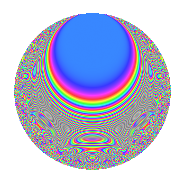# Properties

 Label 804.2.j.bLevel 804 Weight 2 Character orbit 804.j Analytic conductor 6.420 Analytic rank 0 Dimension 68 CM No

# Related objects

## Newspace parameters

 Level: $$N$$ = $$804 = 2^{2} \cdot 3 \cdot 67$$ Weight: $$k$$ = $$2$$ Character orbit: $$[\chi]$$ = 804.j (of order $$6$$ and degree $$2$$)

## Newform invariants

 Self dual: No Analytic conductor: $$6.41997232251$$ Analytic rank: $$0$$ Dimension: $$68$$ Relative dimension: $$34$$ over $$\Q(\zeta_{6})$$ Sato-Tate group: $\mathrm{SU}(2)[C_{6}]$

## $q$-expansion

The dimension is sufficiently large that we do not compute an algebraic $$q$$-expansion, but we have computed the trace expansion.

 $$\operatorname{Tr}(f)(q) =$$ $$68q + 68q^{3} - 2q^{4} - 4q^{7} + 6q^{8} + 68q^{9} + O(q^{10})$$ $$\operatorname{Tr}(f)(q) =$$ $$68q + 68q^{3} - 2q^{4} - 4q^{7} + 6q^{8} + 68q^{9} - 6q^{10} - 2q^{12} + 6q^{13} + 10q^{14} - 2q^{16} - 12q^{20} - 4q^{21} - 22q^{22} + 6q^{24} - 68q^{25} - 19q^{26} + 68q^{27} - 7q^{28} - 8q^{29} - 6q^{30} - 2q^{31} + 15q^{32} - 2q^{36} + 12q^{37} + 4q^{38} + 6q^{39} + 18q^{40} + 10q^{42} + 4q^{43} - 5q^{44} + 16q^{46} - 2q^{48} - 46q^{49} + 27q^{50} + 28q^{52} - 17q^{56} - 4q^{58} - 12q^{60} + 6q^{61} - 34q^{62} - 4q^{63} + 16q^{64} - 22q^{66} + 18q^{67} + 34q^{68} - 56q^{70} + 36q^{71} + 6q^{72} + 6q^{73} + 11q^{74} - 68q^{75} + 14q^{76} - 4q^{77} - 19q^{78} - 6q^{79} - 25q^{80} + 68q^{81} - 26q^{82} - 12q^{83} - 7q^{84} - 33q^{86} - 8q^{87} + 22q^{88} - 6q^{90} + 10q^{92} - 2q^{93} + 16q^{94} - 20q^{95} + 15q^{96} + 18q^{97} - 32q^{98} + O(q^{100})$$

## Embeddings

For each embedding $$\iota_m$$ of the coefficient field, the values $$\iota_m(a_n)$$ are shown below.

For more information on an embedded modular form you can click on its label.

Label $$a_{2}$$ $$a_{3}$$ $$a_{4}$$ $$a_{5}$$ $$a_{6}$$ $$a_{7}$$ $$a_{8}$$ $$a_{9}$$ $$a_{10}$$
499.1 −1.41416 + 0.0125113i 1.00000 1.99969 0.0353860i 0.567637i −1.41416 + 0.0125113i 1.03281 1.78888i −2.82743 + 0.0750601i 1.00000 −0.00710190 0.802729i
499.2 −1.41401 + 0.0238445i 1.00000 1.99886 0.0674328i 4.20985i −1.41401 + 0.0238445i −2.11068 + 3.65581i −2.82481 + 0.143013i 1.00000 0.100382 + 5.95277i
499.3 −1.37740 0.320587i 1.00000 1.79445 + 0.883153i 0.264773i −1.37740 0.320587i 0.135503 0.234697i −2.18854 1.79173i 1.00000 0.0848830 0.364698i
499.4 −1.32266 + 0.500561i 1.00000 1.49888 1.32415i 3.14328i −1.32266 + 0.500561i 2.02612 3.50935i −1.31969 + 2.50168i 1.00000 1.57340 + 4.15750i
499.5 −1.28998 + 0.579621i 1.00000 1.32808 1.49540i 0.235101i −1.28998 + 0.579621i −1.44937 + 2.51039i −0.846427 + 2.69881i 1.00000 −0.136269 0.303274i
499.6 −1.09599 + 0.893755i 1.00000 0.402402 1.95910i 0.0336703i −1.09599 + 0.893755i −0.290483 + 0.503131i 1.30993 + 2.50681i 1.00000 0.0300931 + 0.0369025i
499.7 −1.06182 0.934096i 1.00000 0.254930 + 1.98369i 3.05041i −1.06182 0.934096i 1.90650 3.30215i 1.58226 2.34445i 1.00000 2.84938 3.23900i
499.8 −1.05726 0.939254i 1.00000 0.235605 + 1.98607i 2.76718i −1.05726 0.939254i −2.08271 + 3.60736i 1.61633 2.32109i 1.00000 2.59908 2.92563i
499.9 −1.02913 0.969995i 1.00000 0.118221 + 1.99650i 4.16950i −1.02913 0.969995i 0.853071 1.47756i 1.81493 2.16934i 1.00000 −4.04439 + 4.29096i
499.10 −0.893407 + 1.09628i 1.00000 −0.403650 1.95884i 3.70048i −0.893407 + 1.09628i −0.353219 + 0.611794i 2.50806 + 1.30753i 1.00000 −4.05676 3.30603i
499.11 −0.883912 + 1.10395i 1.00000 −0.437400 1.95158i 1.98108i −0.883912 + 1.10395i 0.674999 1.16913i 2.54107 + 1.24216i 1.00000 2.18701 + 1.75110i
499.12 −0.722396 1.21579i 1.00000 −0.956289 + 1.75656i 1.43594i −0.722396 1.21579i −1.39755 + 2.42063i 2.82643 0.106288i 1.00000 −1.74580 + 1.03732i
499.13 −0.618978 1.27156i 1.00000 −1.23373 + 1.57414i 0.967619i −0.618978 1.27156i −0.571559 + 0.989969i 2.76526 + 0.594407i 1.00000 −1.23039 + 0.598935i
499.14 −0.431235 + 1.34686i 1.00000 −1.62807 1.16163i 2.35066i −0.431235 + 1.34686i −1.38486 + 2.39864i 2.26663 1.69186i 1.00000 3.16602 + 1.01369i
499.15 −0.310623 + 1.37968i 1.00000 −1.80703 0.857120i 2.13147i −0.310623 + 1.37968i 1.61812 2.80266i 1.74385 2.22688i 1.00000 −2.94075 0.662085i
499.16 −0.168883 1.40409i 1.00000 −1.94296 + 0.474254i 3.15821i −0.168883 1.40409i −0.0286491 + 0.0496217i 0.994029 + 2.64800i 1.00000 4.43442 0.533366i
499.17 −0.0466206 + 1.41344i 1.00000 −1.99565 0.131791i 1.77165i −0.0466206 + 1.41344i −0.297950 + 0.516065i 0.279318 2.81460i 1.00000 −2.50413 0.0825955i
499.18 0.0527680 1.41323i 1.00000 −1.99443 0.149147i 0.208091i 0.0527680 1.41323i −1.64198 + 2.84399i −0.316021 + 2.81072i 1.00000 0.294080 + 0.0109805i
499.19 0.143456 1.40692i 1.00000 −1.95884 0.403662i 3.87493i 0.143456 1.40692i 0.231804 0.401496i −0.848927 + 2.69802i 1.00000 −5.45171 0.555882i
499.20 0.315172 1.37865i 1.00000 −1.80133 0.869020i 2.49461i 0.315172 1.37865i 1.03268 1.78865i −1.76580 + 2.20951i 1.00000 3.43919 + 0.786230i
See all 68 embeddings
 $$n$$: e.g. 2-40 or 990-1000 Embeddings: e.g. 1-3 or 775.34 Significant digits: Format: Complex embeddings Normalized embeddings Satake parameters Satake angles

## Inner twists

This newform does not have CM; other inner twists have not been computed.

## Hecke kernels

This newform can be constructed as the kernel of the linear operator $$T_{7}^{68} + \cdots$$ acting on $$S_{2}^{\mathrm{new}}(804, [\chi])$$.